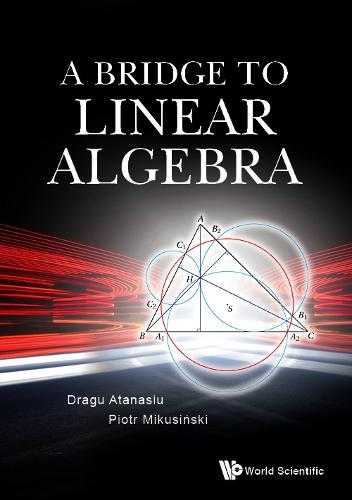•# Bridge To Linear Algebra, A (Hardback)

(author), (author)
£130.00
Hardback 508 Pages / Published: 30/05/2019
• We can order this

Usually dispatched within 10 working days

'The last section is an interesting collection of geometry problems and their solutions from various International Mathematics Olympics ... There are a sufficient number of exercises at the end of each chapter, and the answers to half of them are included at the end of the book, with an occasional full solution here and there. The book prepares the reader for a traditional introductory textbook in linear algebra.'CHOICEThe book makes a first course in linear algebra more accessible to the majority of students and it assumes no prior knowledge of the subject. It provides a careful presentation of particular cases of all core topics. Students will find that the explanations are clear and detailed in manner. It is considered as a bridge over the obstacles in linear algebra and can be used with or without the help of an instructor.While many linear algebra texts neglect geometry, this book includes numerous geometrical applications. For example, the book presents classical analytic geometry using concepts and methods from linear algebra, discusses rotations from a geometric viewpoint, gives a rigorous interpretation of the right-hand rule for the cross product using rotations and applies linear algebra to solve some nontrivial plane geometry problems.Many students studying mathematics, physics, engineering and economics find learning introductory linear algebra difficult as it has high elements of abstraction that are not easy to grasp. This book will come in handy to facilitate the understanding of linear algebra whereby it gives a comprehensive, concrete treatment of linear algebra in R(2) and R(3). This method has been shown to improve, sometimes dramatically, a student's view of the subject.

Publisher: World Scientific Publishing Co Pte Ltd
ISBN: 9789811200229
Number of pages: 508